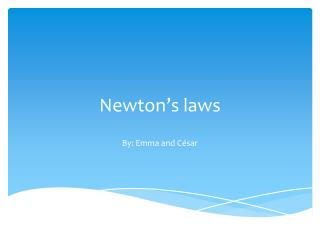DownloadDownload PresentationNewton’s laws

Newton’s laws

Télécharger la présentationNewton’s laws

- - - - - - - - - - - - - - - - - - - - - - - - - - - E N D - - - - - - - - - - - - - - - - - - - - - - - - - - -
Presentation Transcript

1. Newton’s laws By: Emma and César

2. Newton’s First Law Of Motion Definition: Every object in a state of uniform motion tends to remain in that state of motion unless an external force is applied to it.

3. Activity 1: Body At Rest In this experiment you will learn about inertia. In it, you will try to remove a card board from under an object without moving the coin on top.

4. MaterialsYou will need the following items for this experiment A cup A coin A piece of card boardProcedure1. Place the piece of card board on top of a cup.2. Put the coin on top of the piece of card board.3. Quickly (and in one smooth motion) flick the piece of card board from between the coin and cup.4. Write down what happens.

5. Activities2:And they are off !!! In this experiment you will discover how Newton’s First Law works by conducting a race with two jars.

6. MaterialsYou will need the following items for this experiment:• two identical jars with lids (either plastic or glass jars)• flour or sand to fill one of the jars• iron filings or small lead pellets to fill one of the jars• two identical, empty three-ring binders (at least 2.5” in width)• a measuring tape

7. Procedure 1. Fill one jar with flour or sand. Pack it tightly.2. Fill the other jar with iron filings or small lead pellets. Again, fill it tightly.3. Put lids on both of the jars. Lids should be on tight.4. Place both three-ring binders next to each other on a wooden or tile floor. Place each jar on its side and release both from the top of the “ramps” at exactly the same time.5. In the Table below, record how far each jar rolled. Do not measure the binder itself, just the distance from the end of the binder to where each jar actually stopped.6. Repeat Steps 3-4 for each of the surfaces listed on the Table. 7. Fill in the Table with your results for each race.

8. Newton’s Second Law Of Motion Definition :second law of motion the rate of change of momentum is proportional to the imposed force and goes in the direction of the force

9. Activity 1: pulling car Pass a piece of string with a mass hanging on one end over a pulley. Attach the other end to the trolley so that, when the mass is released, it causes the trolley to accelerate. Choose a length of string which allows the mass to touch the ground when the trolley is about two thirds along the bench length.  Fit the trolley with a double segment black card. Clamp the light gate at a height to allow both segments of the card to interrupt the light beam when the trolley moves through the gate. Measure the width of each segment with a ruler and enter the values into the data-logging software. Connect the light gate via an interface to a computer programmed.Configure the program to obtain measurements of acceleration derived from the double interruptions of the light beam by the card. The program will require the dimensions of the card. The internal calculation within it involves using the interruption times for the two segments to obtain two velocities. The difference between these divided by the time between them yields the acceleration.

10. Procedure Data collection A:Pull the trolley back so that the mass is raised to just below the pulley. Position the light gate so that it will detect the motion of the trolley soon after it has started moving. Set the software to record data. Release the trolley. Observe the measurement for the acceleration of the trolley. B:Move the light gate about 20 cm further along the table. Repeat the measurement, releasing the trolley from the same point as before. Observe the we measurement and compare it with the first. C:Repeat this process several times until the light gate is as near as it can be to the pulley, while still allowing the trolley to pass through.

11. Activity2: Pulling Notice that doubling the force by adding another dog doubles the acceleration. Oppositely, doubling the mass to 100 kg would halve the acceleration to 2 m/s2. If two dogs are on each side, then the total force pulling to the left (200 N) balances the total force pulling to the right (200 N). That means the net force on the sled is zero, so the sled doesn’t move. If you want to calculate the acceleration, first you need to modify the force equation to get a = F/m. When you plug in the numbers for force (100 N) and mass (50 kg), you find that the acceleration is 2 m/s2.

12. Newton's Third Law Of Motion

13. Definition Newtons third law of motion: